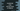# JavaScript map with an array of objects## Example of JavaScript map method with an array of objects:

The JavaScript map method is used to call a function on each element of an array to create a different array based on the outputs of the function. It creates a new array without modifying the elements of the original array.

In this tutorial, I will show you how to use this map method with an array of objects with an example.

### Syntax of map:

The syntax of JavaScript map method is defined as like below :

``let finalArray = arr.map(callback( currentValue[, index[, array]]), arg)``

Following are the meanings of these parameters :

• callback: This is the callback method. It is called for each of the array arr elements. The returned value of this method is added to the final array finalArray.
• currentValue: The current array element.
• index: This is the index of the current element. It is an optional value.
• array: The given array. This is also optional.
• arg: This is also an optional value. It is used as the this value. If it is empty, undefined is passed.

The return value of this method is a new array with elements created by using the callback method.

## Example of map with a simple array :

Let me show you one simple example of map() :

``````let givenArr = ['one', 'two', 'three', 'four'];

let lengthArr = givenArr.map(item => item.length);

console.log(lengthArr);``````

Here,

• givenArr is an array of strings.
• We are using map on this array. The callback method converts each item to its length i.e. the result array will hold the length of each word of givenArr.

It will print the below output :

``[ 3, 3, 5, 4 ]``

## Example of map with an array of objects :

Similar to the above example, we can also use map with an array of objects :

``````let studentArr = [
{
id: 1,
name: "Alex",
age: 20,
marks: 40,
isAvailable: false,
},
{
id: 2,
name: "Bob",
age: 22,
marks: 40,
isAvailable: true,
},
{
id: 3,
name: "Chandler",
age: 21,
marks: 40,
isAvailable: false,
},
{
id: 4,
name: "Daisy",
age: 19,
marks: 40,
isAvailable: true,
},
];

let newArray = studentArr.map((item) => ({ name: item.name, age: item.age }));

console.log(newArray);``````

In this example :

1. We have defined one array with different student objects, studentArr
2. This array holds the information of students with different values. Suppose, we need to filter out these objects with only name and age for each. We can do that using map as showing above.
3. The callback method creates a new object with only name and age of each values.

This program will print the below output :

``````[
{ name: 'Alex', age: 20 },
{ name: 'Bob', age: 22 },
{ name: 'Chandler', age: 21 },
{ name: 'Daisy', age: 19 }
]``````